Question

A 16.1 mL sample of a 0.441 M aqueous hydrocyanic acid solution is titrated with a 0.348 M aqueous potassium hydroxide solution. What is the pH at the start of the titration, before any potassium hydroxide has been added?

pH = ?

We need at least 10 more requests to produce the answer.

0 / 10 have requested this problem solution

The more requests, the faster the answer.

All students who have requested the answer will be notified once they are available.

#### Earn Coins

Coins can be redeemed for fabulous gifts.

Similar Homework Help Questions
• ### A 17.0 mL sample of a 0.357 M aqueous acetic acid solution is titrated with a...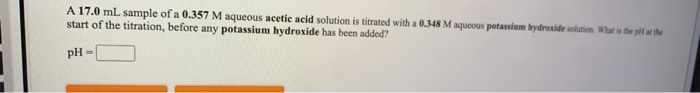A 17.0 mL sample of a 0.357 M aqueous acetic acid solution is titrated with a 0.348 M aqueous potassium hydroxide solution. What is the pll at the start of the titration, before any potassium hydroxide has been added? pH-

• ### When a 21.4 mL sample of a 0.311 M aqueous hydrocyanic acid solution is titrated with...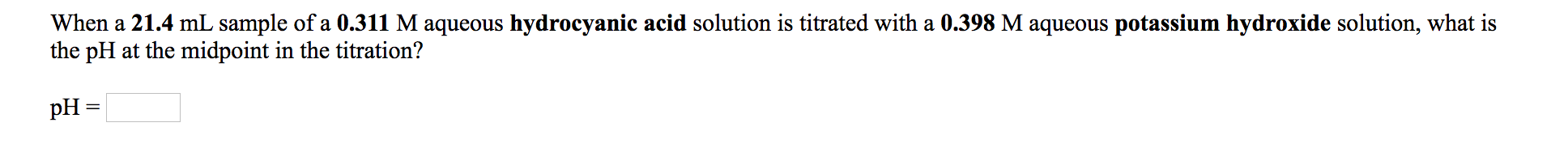When a 21.4 mL sample of a 0.311 M aqueous hydrocyanic acid solution is titrated with a 0.398 M aqueous potassium hydroxide solution, what is the pH at the midpoint in the titration? pH = A 45.3 mL sample of a 0.370 M aqueous acetic acid solution is titrated with a 0.424 M aqueous potassium hydroxide solution. What is the pH after 23.1 mL of base have been added? pH =

• ### When a 19.8 mL sample of a 0.460 M aqueous acetic acid solution is titrated with...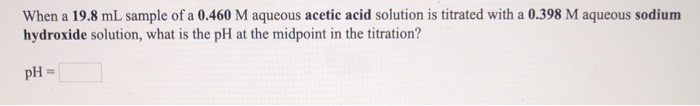When a 19.8 mL sample of a 0.460 M aqueous acetic acid solution is titrated with a 0.398 M aqueous sodium hydroxide solution, what is the pH at the midpoint in the titration? pH = A 17.5 mL sample of a 0.430 M aqueous hydrofluoric acid solution is titrated with a 0.381 M aqueous potassium hydroxide solution. What is the pH at the start of the titration, before any potassium hydroxide has been added? pH-

• ### a. When a 20.0 mL sample of a 0.431 M aqueous nitrous acid solution is titrated...

a. When a 20.0 mL sample of a 0.431 M aqueous nitrous acid solution is titrated with a 0.369 M aqueous sodium hydroxide solution, what is the pH at the midpoint in the titration? b. A 26.4 mL sample of a 0.351 M aqueous nitrous acid solution is titrated with a 0.345 M aqueous potassium hydroxide solution. What is the pH at the start of the titration, before any potassium hydroxide has been added?

• ### When a 15.6 mL sample of a 0.328 M aqueous hydrocyanic acid solution is titrated with...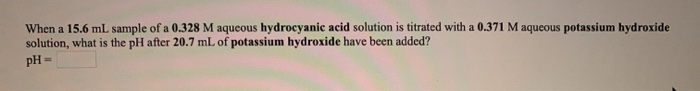When a 15.6 mL sample of a 0.328 M aqueous hydrocyanic acid solution is titrated with a 0.371 M aqueous potassium hydroxide solution, what is the pH after 20.7 mL of potassium hydroxide have been added? pH

• ### A 26.0 mL sample of a 0.462 M aqueous nitrous acid solution is titrated with a 0.363 M aqueous potassium hydroxide solut...

A 26.0 mL sample of a 0.462 M aqueous nitrous acid solution is titrated with a 0.363 M aqueous potassium hydroxide solution. What is the pH at the start of the titration, before any potassium hydroxide has been added?

• ### A 23.0 mL sample of a 0.497 M aqueous acetic acid solution is titrated with a...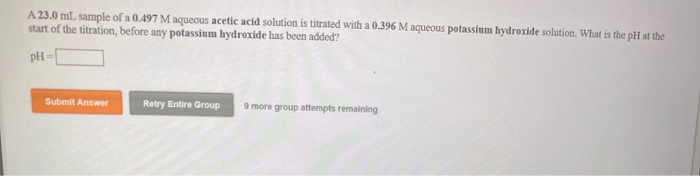A 23.0 mL sample of a 0.497 M aqueous acetic acid solution is titrated with a 0.396 M aqueous potassium hydroxide solution. What is the pH at the start of the titration, before any potassium hydroxide has been added? PH Submit Answer Retry Entire Group 9 more group attempts remaining What is the pH at the equivalence point in the titration of a 25.5 mL sample of a 0.411 M aqueous acetic acid solution with a 0.352 M aqueous barium...

• ### 1. When a 21.5 mL sample of a 0.329 M aqueous hypochlorous acid solution is titrated...

1. When a 21.5 mL sample of a 0.329 M aqueous hypochlorous acid solution is titrated with a 0.456 M aqueous potassium hydroxide solution, what is the pH after 23.3 mL of potassium hydroxide have been added? pH = 2. When a 16.0 mL sample of a 0.404 M aqueous hydrocyanic acid solution is titrated with a 0.418 M aqueous barium hydroxide solution, what is the pH at the midpoint in the titration? pH =

• ### When a 23.2 mL sample of a 0.345 M aqueous acetic acid solution is titrated with...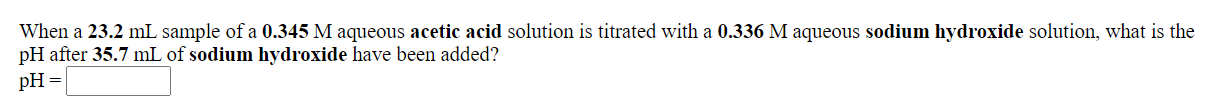When a 23.2 mL sample of a 0.345 M aqueous acetic acid solution is titrated with a 0.336 M aqueous sodium hydroxide solution, what is the pH after 35.7 mL of sodium hydroxide have been added? pH = What is the pH at the equivalence point in the titration of a 27.6 mL sample of a 0.423 M aqueous hydrocyanic acid solution with a 0.431 M aqueous potassium hydroxide solution? pH=

• ### When a 17.3 mL sample of a 0.447 M aqueous hydrocyanic acid solution is titrated with...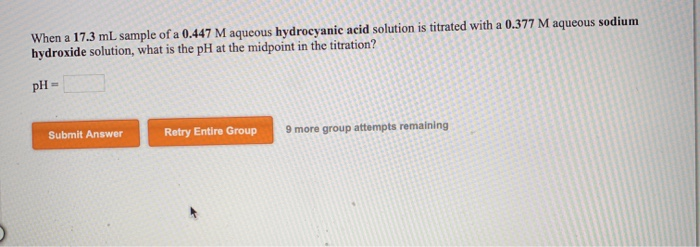When a 17.3 mL sample of a 0.447 M aqueous hydrocyanic acid solution is titrated with a 0.377 M aqueous sodium hydroxide solution, what is the pH at the midpoint in the titration? pH = Submit Answer Retry Entire Group 9 more group attempts remaining A 36.4 mL sample of a 0.391 M aqueous hydrofluoric acid solution is titrated with a 0.250 M aqueous sodium hydroxide solution. What is the pH after 17.9 mL of base have been added? pH...